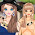### Bankers Algorithm- Systems Lab - C Program

//Bankers Algorithm

Program :

#include<stdio.h>
#define true 1
#define false 0
int m,n,i,j,count=0,process;
int max,alloc,need,c,avail,finish;
{
for(i=0;i<m;i++)
for(j=0;j<n;j++)
scanf("%d",&t[i][j]);
}
void printtable(int t)
{
for(i=0;i<m;i++)
{
for(j=0;j<n;j++)
printf("\t%d",t[i][j]);
printf("\n");
}
}
{
for(j=0;j<n;j++)
scanf("%d",&v[j]);
}
void printvector(int v)
{
for(j=0;j<n;j++)
printf("\t%d",v[j]);
}
void init()
{
printf("enter the number of process\n");
scanf("%d",&m);
printf("enter the number of resources\n");
scanf("%d",&n);
printf("enter the claim table\n");
printf("enter the allocation table\n");
printf("enter the max units of each resource\n");
for(i=0;i<n;i++)
finish[i]=false;
}

void findavail()
{
int sum;
for(j=0;j<n;j++)
{
sum=0;
for(i=0;i<m;i++)
{
sum=sum+alloc[i][j];
}
avail[j]=c[j]-sum;
}
}
void findneed()
{
for(i=0;i<m;i++)
{
for(j=0;j<n;j++)
{
need[i][j]=max[i][j]-alloc[i][j];
}

}
}
void selectprocess()
{
int flag;
for(i=0;i<m;i++)
{
for(j=0;j<n;j++)
{
if(need[i][j]<=avail[j])
flag=1;
else
{
flag=0;
break;
}
}
if((flag==1)&&(finish[i]==false))
{
process=i;
count++;
break;
}
}
printf("current status is\n");
printtable(alloc);
if(flag==0)
{
printf("system is in unsafe state\n");
exit(1);
}
printf("system is in safe state");
}
void executeprocess(int p)
{
printf("excuting process is %d",p);
printtable(alloc);
}
void releaseresource()
{
for(j=0;j<n;j++)
avail[j]=avail[j]+alloc[process][j];
for(j=0;j<n;j++)
{
alloc[process][j]=0;
need[process][j]=0;
}
}

main()
{
init();
findavail();
findneed();
do
{
selectprocess();
finish[process]=true;
executeprocess(process);
releaseresource();
}while(count<m);
printf("\n all proces executed correctly");
}

1.2.thnx....

3.4.thanqqqq uuu so muchh luv :*

5.what the hell is this.........

6.Detailed program visit

proud2bengineer.blogspot.com

7.8.9.thnqqqqqqqqqqqqqqq
bosssssssssss

10.chothe it is!!!!!!!!!!!!!

11.thnku :) :) :)

12.vandanegalu :))

13.Detailed program visit
proud2bengineer.com

14.thank you

15.16.Great! i like your post so much stick-rpg2.com

17.great article, I was very impressed about it, wish you would have stayed next share

www.appbaixar.com

18.I was very impressed by this post, this site has always been pleasant news. Thank you very much for such an interesting post. Keep working, great job! In my free time, I like play game: mutilateadoll2game.com. What about you?

19.Alot of blogs I see these days don't really provide anything that I'm interested in, but I'm most definately interested in this one. Just thought that I would post and let you know. Nice! thank you so much! Thank you for sharing.
www.baixar-whatsapp-gratis.com.br